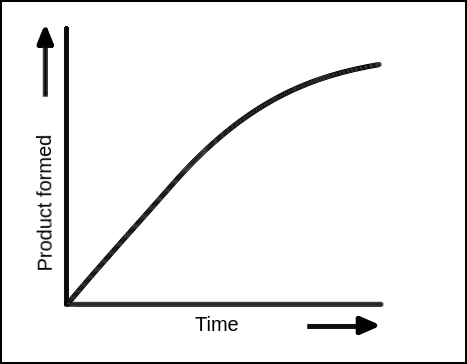During the course of an enzymatic reaction, [S] decreases, and [P] increases. This results in an overall decrease in the reaction rate, as illustrated in Figure 1. To measure the reaction rate of an enzymatic reaction corresponding to a specific substrate concentration, it is, therefore, necessary to measure the reaction rate at the beginning of the reaction. At the beginning of the reaction, the substrate concentration can be assumed to be constant, and the progress curve is close to linear. Thus, the rate of the reaction, which is equal to the slope of the progress curve, can be obtained using linear regression. The reaction rate at the beginning of the reaction is called the initial reaction rate or V0 .Figure 1: Progress curve of a general enzymatic reaction.

1. Atkins, Peter W.; de Paula, Julio; Friedman, Ronald (2009). Quanta, Matter, and Change: A molecular approach to physical chemistry. Oxford University Press. ISBN 978-0-19-920606-3.

2. Lehninger, Albert L.; Nelson, David L.; Cox, Michael M. (2008). Principles of Biochemistry (5th ed.). New York, NY: W.H. Freeman and Company. ISBN 978-0-7167-7108-1.

Km

Theory overview